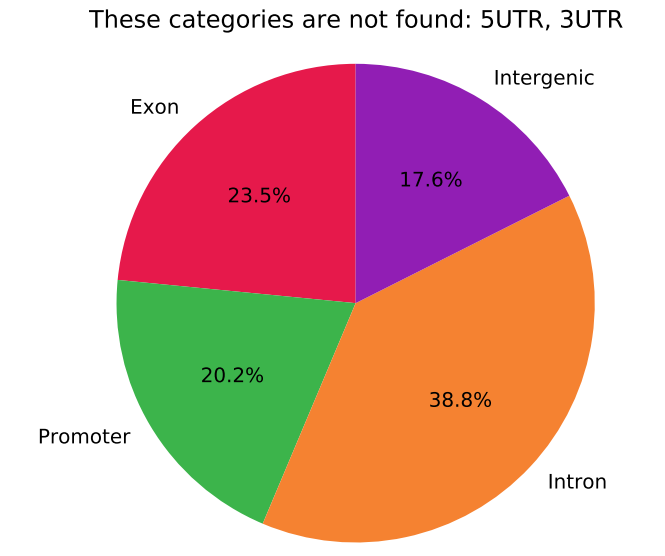How to plot/combine biological replicates on a genomic features plot for methylated array?
1
0
Entering edit mode
14 months ago
Pratik ▴ 810

Hello Biostars Community,

How would I plot biological replicates on a genomic features plot for methylated array?

Should I plot the mean from all the samples for each probe? or would it be better to plot each biological replicate separately? Hoping there is a way to combine them so it's concise in a way?

I want to plot figures like this: What cut-offs to use for Genomic Features Pie Chart for Methylation Array Data?Thank you in advance : )

array methylation beadchip plot features genomic • 692 views
2
Entering edit mode
14 months ago
Papyrus ★ 2.1k

This depends a lot on your goal. If you just want to show, across your data in general (or maybe between different groups), how methylation is distributed across genomic features, I would pool the information on the biological replicates, yes. But it is not necessary to do any operation (like you suggested, means across each CpG) for the plots. Simply concatenate all the data for the replicates. For example, using ggplot2 in a tidy data format, you will have to input something like this:

cpg sample  value   genomic_feature
cg1 A   0.2 promoter
cg2 A   0.8 exon
cg3 A   0.1 intergenic
cg1 B   0.3 promoter
cg2 B   0.9 exon
cg3 B   0.2 intergenic
…   …   …   …


Thus, when representing the boxplots, violinplots, separating by genomic feature, the data across the replicates will be pooled

0
Entering edit mode

I really appreciate you going above and beyond to help me Papyrus! I have a couple different groups and each group has more than a couple biological replicates. Is mean/average a good way to pool together the biological replicates? Or is there a better way?

Thank you again. Really looking forward to your response!

0
Entering edit mode

You can take the mean/average to pool the replicates, and it is OK. Nonetheless, you have another option which does not involve losing/pooling information across the replicates. As I said, if you directly input all the replicate points (without taking the mean) into the boxplot/violin plots, the results should be pretty similar, because you have many CpGs and most are correlated between your replicates. You can check the two approaches.

Try this example in R:

library(ggplot2)

# Example input data

# Create methylation values
sample1 <- rbeta(10000, shape1 = 0.2, shape2 = 0.2)
sample2 <- sample1 + rnorm(10000,0,0.01)
sample2[sample2 > 1] <- 1
sample2[sample2 < 0] <- 0

data <- data.frame(
cpg = rep(paste0("cg",1:10000),2),
sample = c(rep("A",10000),rep("B",10000)),
value = c(sample1,sample2),
genomic_feature = sample(c("promoter","exon","intergenic"),20000,replace = T)
)

# Plot
ggplot(data,aes(x = genomic_feature, y = value)) + geom_violin() + geom_boxplot(width = 0.2)

# Take the mean across replicates
data2 <- data[1:10000,]
data2$value <- (data$value[1:10000] + data$value[10001:20000]) / 2 # Plot ggplot(data2,aes(x = genomic_feature, y = value)) + geom_violin() + geom_boxplot(width = 0.2) # And for pie charts: example "low" methylation CpGs ggplot(data[data$value <= 0.2,],aes(x = factor(1), fill = genomic_feature)) + geom_bar(width = 1) + coord_polar("y")
ggplot(data2[data2\$value <= 0.2,],aes(x = factor(1), fill = genomic_feature)) + geom_bar(width = 1) + coord_polar("y")